Function Repository Resource:

# NSeries

Find a numerical approximation of a series expansion of a function

Contributed by: Wolfram Research
 ResourceFunction["NSeries"][f,{x,x0,n}] gives a numerical approximation to the series expansion of f about the point x=x0 including the terms (x-x0)-n through (x-x0)n.

## Details and Options

The function f must be numeric when its argument x is numeric.
ResourceFunction["NSeries"] will construct standard univariate Taylor or Laurent series.
ResourceFunction["NSeries"] samples f at points on a circle in the complex plane centered at x0 and uses InverseFourier. The option "Radius" specifies the radius of the circle.
The region of convergence will be the annulus (containing the sampled points) where f is analytic.
ResourceFunction["NSeries"] will not return a correct result if the disk centered at x0 contains a branch cut of f.
The result of ResourceFunction["NSeries"] is a SeriesData object.
If the result of ResourceFunction["NSeries"] is a Laurent series, then the SeriesData object is not a correct representation of the series, as higher-order poles are neglected.
No effort is made to justify the precision in each of the coefficients of the series.
ResourceFunction["NSeries"] is unable to recognize small numbers that should in fact be zero. Chop is often needed to eliminate these spurious residuals.
The number of sample points chosen is 2Ceiling[Log[2,n]]+1.
The following options can be given:
 "Radius" 1 radius of circle on which f is sampled WorkingPrecision MachinePrecision precision used in internal computations

## Examples

### Basic Examples (3)

This is a power series for the exponential function around x=0:

 In:=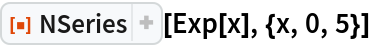Out=Chop is needed to eliminate spurious residuals:

 In:=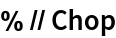Out=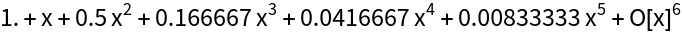Using extended precision may also eliminate spurious imaginaries:

 In:=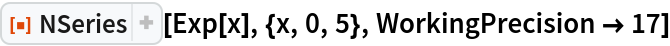Out=### Scope (2)

Find expansions in the complex plane:

 In:=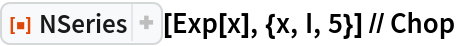Out=Find Laurent expansions about essential singularities:

 In:=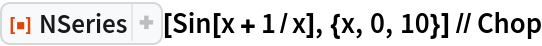Out=Series will not find Laurent expansions about essential singularities:

 In:=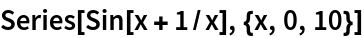Out=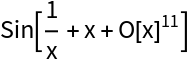### Options (2)

Use "Radius" to pick the annulus within which the Laurent series will converge:

 In:=Out=Laurent series for x3:

 In:=Out=In:=Out=In:=Out=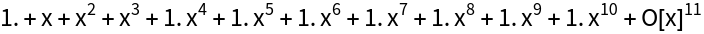### Applications (3)

A function defined only for numerical input:

 In:=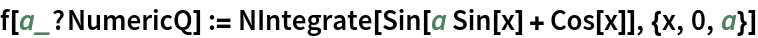Find a series expansion of f:

 In:=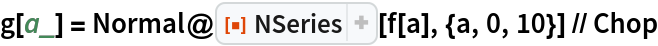Out=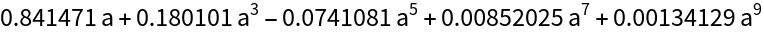Check:

 In:=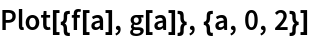Out=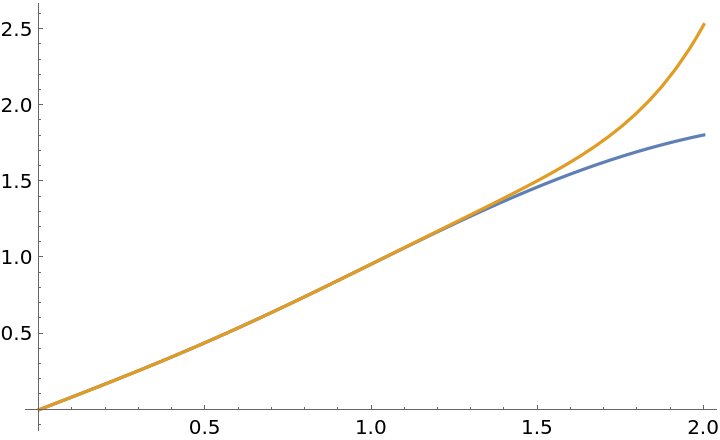### Properties & Relations (2)

NResidue can also be used to construct a series of a numerical function:

 In:=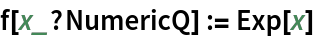In:=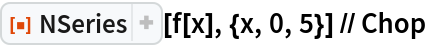Out=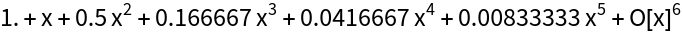Using NResidue:

 In:=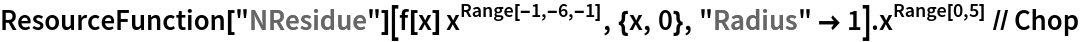Out=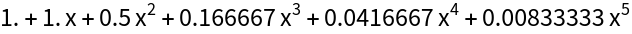### Possible Issues (2)

NSeries can have aliasing problems due to InverseFourier:

 In:=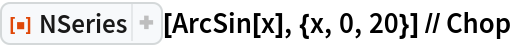Out=The correct expansion is analytic at the origin:

 In:=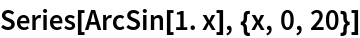Out=SeriesData cannot correctly represent a Laurent series. Here is the square of the series of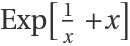:

 In:=Out=Here is the SeriesData representation of the Laurent series of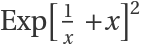:

 In:=Out=### Neat Examples (2)

Find the series expansion of the generating function for unrestricted partitions:

 In:=In:=Out=Check:

 In:=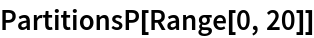Out=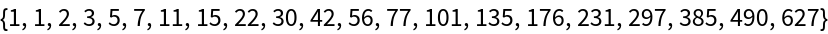## Requirements

Wolfram Language 11.3 (March 2018) or above

## Version History

• 1.0.0 – 13 March 2019